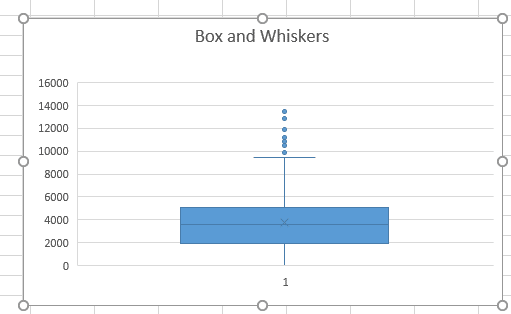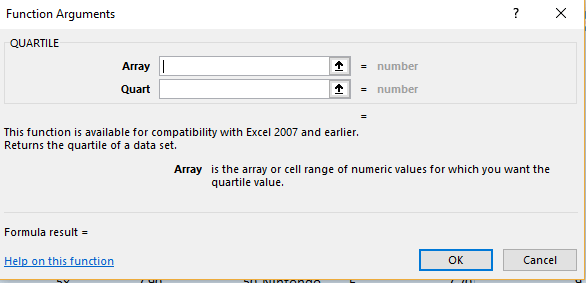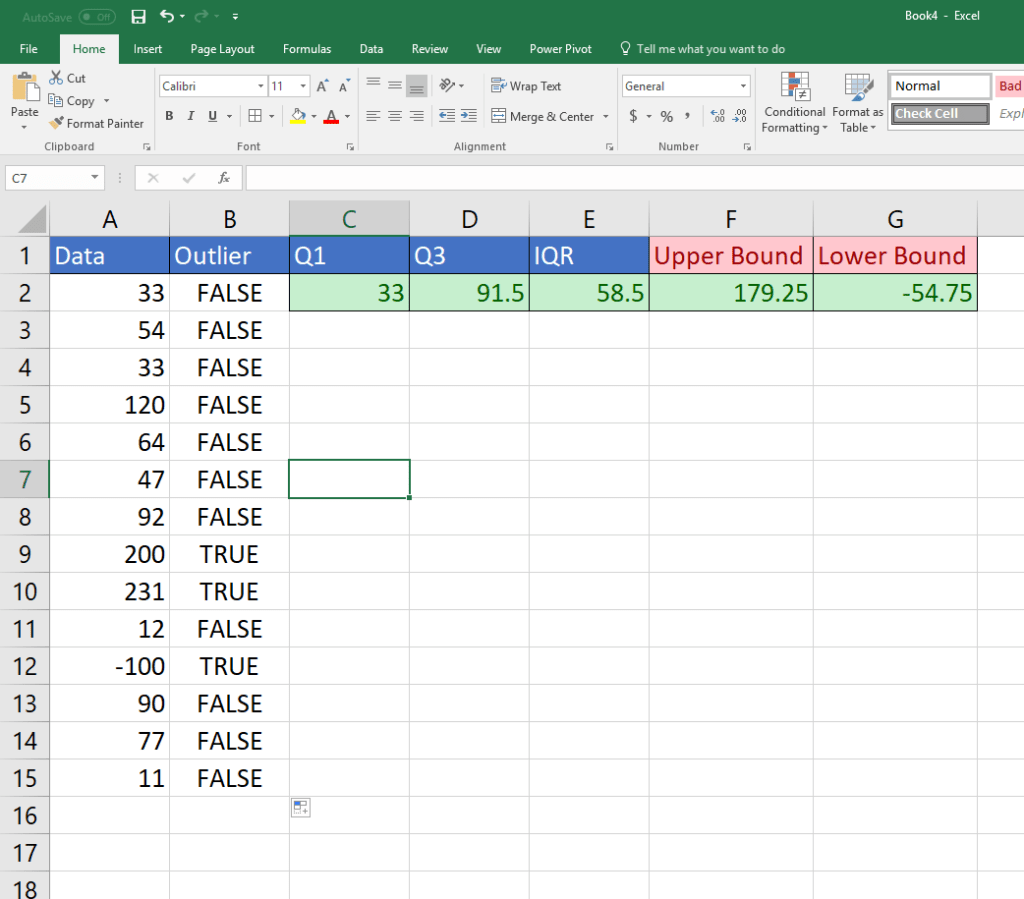# How to Find Outliers in Excel

Excel has a lot of underused function that can greatly improve your data analysis. One of the best features is it’s statistical capabilities So you can find outliers in Excel easily with simple statistics formulas..

Why should you isolate and eliminate outliers in your data? One of the main reasons is that these extreme data points will skew your results often causing misleading assumption. It will essentially drag your average in a positive or negative direction. Removing these outliers will give you a better picture of your data.

You can use both visualizations and formulas to identify outliers in Excel. Let’s get started with some statistics to find an outlier in Excel. See a great Master Excel Beginner to Advanced Course to improve your skills fast.

Here are the statistical concepts that we will employ to find outliers:

1. Box Plots – in the image below you can see that several points exist outside of the box. The box is the central tendency of the data.  It is clustered around a middle value. The upper bound line is the limit of the centralization of that data.
2. Quartiles–  represent how the data is broken up into quarters.### What are Quartiles and How to Use Them

You can easily identify outliers of your data by using Box and Whisker charts. These are plots that show you how data is clustered around a central measure such as the median(middle) value in the data. The quartile is a dividing point which splits the data into quarters. There are 3 quartiles: Q1, Q2, and Q3. The first quartile(Q1) is the lower quarter of your data where lesser values live. The Q2 is the middle value and Q3 is the upper portion of the data. The range of values from Q1 to Q3 are called the inter-quartile range(IQR).

Outliers live outside the inner quartile range. By statistical definition, they live 1.5 times below or above your inner quartile range.

Box and Whisker Plots show you where the data lies in and outside your inner quartile range

Here is the formula you will be utilizing in Excel.

QUARTILE(array, quart)

The two arguments in the functions are the data that you want to explore. in other words, when you have your data in Excel,it is mostly a list of values in a column. This list is your data array. The other part of the arguments is the quartile you want to define. For example, 1, 2, 3.You should identify the 1st and 3rd quartiles by using this formula

How to calculate the inner quartile range. This is calculated by subtracting Q1 from Q3. This can be named IQR
Next you will need to calcuate the upper and lower limit of your range or your data. You can do this by following the formula below:

Lower range limit = Q1 – (1.5* IQR). Essentially this is 1.5 times the inner quartile range subtracting from your 1st quartile.
Higher range limit = Q3 + (1.5*IQR) This is 1.5 times IQR+ quartile 3.

Now if any of your data falls below or above these limits, it will be considered an outlier.